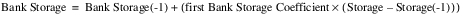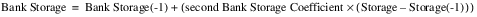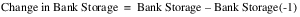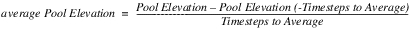Bank Storage
The Bank Storage methods are used to calculate the volume of water stored in the Reservoir banks. These methods also calculate the change in the volume of water stored in the Reservoir banks from one timestep to the next.None
None should be chosen if the user does not want to calculate the amount of Bank Storage in the Reservoir. This is the default method for the Bank Storage category. Bank Storage and the Change in Bank Storage are set to zero but are not displayed. No calculations are performed in this method.
There are no slots specific to this method.Input Bank Storage
The Input Bank Storage method allows users to directly input values into the Bank Storage slot or to set these values using a rule. Change in Bank Storage is calculated internally in RiverWare for use in the mass balance equations.
Slots Specific to This Method
Bank Storage
Type: Series Slot
Units: Volume
Description: Volume of water stored in the reservoir banks
Information:
I/O: Input only
Change in Bank Storage
Type: Series Slot
Units: Volume
Description: change in volume of water stored in the reservoir banks
Information:
I/O: Output onlyCRSS Bank Storage
The CRSS Bank Storage method replicates the U.S. Bureau of Reclamation’s CRSS bank storage calculation. The Bank Storage and the Change in Bank Storage are calculated using the Reservoir Storage and the Bank Storage Coefficients.
Slots Specific to This Method
Bank Storage
Type: Series Slot
Units: Volume
Description: Volume of water stored in the reservoir banks
Information:
I/O: Output only
Bank Storage Coefficient
Type: Table Slot
Units: No Units vs No Units
Description: Gain or loss of storage vs change in bank storage
Information: The first coefficient (column zero) is for increasing storage and the second coefficient is for decreasing storage.
I/O: Required input
Change in Bank Storage
Type: Series Slot
Units: Volume
Description: Change in volume of water stored in the reservoir banks
Information:
I/O: Output only
Method Details
There are two ways Bank Storage can be calculated depending on the current Storage of the Reservoir. If the Reservoir’s current Storage is greater than the Reservoir’s Storage at the previous timestep, the Storage is increasing. Bank Storage is calculated using the following equation:If the Reservoir’s current Storage is less than the Reservoir’s Storage at the previous timestep, the Storage is decreasing. Bank Storage is calculated using the following equation:The Change in Bank Storage is calculated using the following equation regardless of which method was used to compute Bank Storage.where:
– Bank Storage = the volume of water stored in the banks of the Reservoir at the current timestep
– Bank Storage(-1) = the volume of the water stored in the banks of the Reservoir at the previous timestep.
– Storage = the volume of water in the Reservoir at the current timestep
– Storage(-1) = the volume of water in the Reservoir at the previous timestepAverage Stage Change
The Average Stage Change method calculates the Bank Storage and Change in Bank Storage based on the flow from storage. The flow from storage is a function of the average stage change over a user defined number of timesteps.
Slots Specific to This Method
Ave Stage Change Coeffs
Type: Table Slot
Units: Area per Time and Flow
Description: Coefficient describing flow for a given change in pool elevation and a constant representing flow from bank storage
Information:
I/O: Required input
Bank Storage
Type: Series Slot
Units: Volume
Description: Volume of water stored in the reservoir banks
Information:
I/O: Output only
Change in Bank Storage
Type: Series Slot
Units: Volume
Description: Change in volume of water stored in the reservoir banks
Information:
I/O: Output only
Timesteps to Average
Type: Table Slot
Units: No Units
Description: Number of timesteps used to calculate average pool elevation.
Information:
I/O: Required input
Method Details
The average stage change is calculated using the following equation:The change in flow to bank storage is calculated using the following equation:The flow is converted to a volume by multiplying the value by the current timestep. The Change in Bank Storage is calculated using the following equation:Revised: 08/02/2021